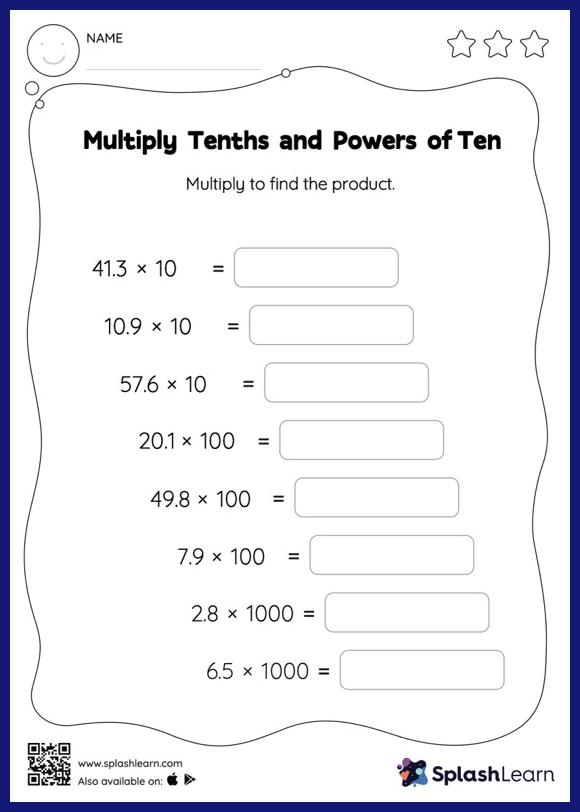# Multiply Tenths and Powers of Ten: Horizontal Multiplication Worksheet

Home > Multiply Tenths and Powers of Ten: Horizontal MultiplicationThis worksheet strengthens students' accuracy and strategic flexibility while they multiply tenths and powers of ten. Multiplying a decimal by a power of 10 moves the decimal point to the right by the same amount as the number of zeros in the power of 10. Students practice this concept extensively in multiply tenths and powers of ten worksheet. In this worksheet, students practice solving problems written in the horizontal format. How numbers are laid out in a problem affects the method a student employs to solve it. Therefore to develop actual fluency and mastery of multiple strategies, students must practice different formats.